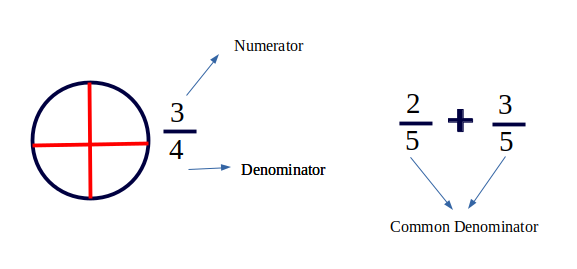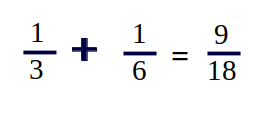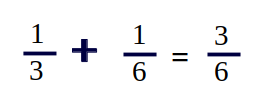Skip to content
Related Articles
Least Common Denominator (LCD)
• Difficulty Level : Easy
• Last Updated : 01 Apr, 2021

The lowest Common Denominator or Least Common Denominator is the Least Common Multiple of the denominators of a set of fractions.Common denominator : when the denominators of two or more fractions are the same.
Least Common denominator is the smallest of all common denominators.
Why do we need LCD
It simplifies addition, subtraction and comparing fraction.
Common Denominator can be simply evaluated by multiplying the denominators. In this case, 3 * 6 = 18But that may not always be least common denominator, as in this case LCD = 6 and not 18. LCD is actually LCM of denominators.
Examples :

```LCD for fractions 5/12 and 7/15 is 60.
We can write both fractions as 25/60 and
28/60 so that they can be added and
subtracted easily.

LCD for fractions 1/3 and 4/7 is 21.```Example Problem : Given two fractions, find their sum using least common dominator.
Examples

```Input :  1/6  +  7/15
Output : 19/30
Explanation : LCM of 6 and 15 is 30.
So, 5/30  +  14/30 = 19/30
Input :  1/3  +  1/6
Output : 3/6
Explanation : LCM of 3 and 6 is 6.
So, 2/6  +  1/6 = 3/6```

Note* These answers can be further simplified by Anomalous cancellation.

## C++

 `// C++ Program to determine``// LCD of two fractions and``// Perform addition on fractions``#include ``using` `namespace` `std;` `// function to calculate gcd``// or hcf of two numbers.``int` `gcd(``int` `a, ``int` `b)``{``    ``if` `(a == 0)``        ``return` `b;``    ``return` `gcd(b % a, a);``}` `// function to calculate``// lcm of two numbers.``int` `lcm(``int` `a, ``int` `b)``{``    ``return` `(a * b) / gcd(a, b);``}` `void` `printSum(``int` `num1, ``int` `den1,``              ``int` `num2, ``int` `den2)``{``    ``// least common multiple``    ``// of denominators LCD``    ``// of 6 and 15 is 30.``    ``int` `lcd = lcm(den1, den2);` `    ``// Computing the numerators for LCD:``    ``// Writing 1/6 as 5/30 and 7/15 as``    ``// 14/30``    ``num1 *= (lcd / den1);``    ``num2 *= (lcd / den2);` `    ``// Our sum is going to be res_num/lcd``    ``int` `res_num = num1 + num2;``    ``cout << res_num << ``"/"` `<< lcd;``}` `// Driver Code``int` `main()``{``    ``// First fraction is 1/6``    ``int` `num1 = 1, den1 = 6;` `    ``// Second fraction is 7/15``    ``int` `num2 = 7, den2 = 15;` `    ``printSum(num1, den1, num2, den2);``    ``return` `0;``}`

## Java

 `// Java Program to determine LCD of two``// fractions and Perform addition on``// fractions``public` `class` `GFG {``    ` `    ``// function to calculate gcd or``    ``// hcf of two numbers.``    ``static` `int` `gcd(``int` `a, ``int` `b)``    ``{``        ``if` `(a == ``0``)``            ``return` `b;``            ` `        ``return` `gcd(b % a, a);``    ``}``    ` `    ``// function to calculate lcm of``    ``// two numbers.``    ``static` `int` `lcm(``int` `a, ``int` `b)``    ``{``        ``return` `(a * b) / gcd(a, b);``    ``}``    ` `    ``static` `void` `printSum(``int` `num1, ``int` `den1,``                         ``int` `num2, ``int` `den2)``    ``{``        ` `        ``// least common multiple of``        ``// denominators LCD of 6 and 15``        ``// is 30.``        ``int` `lcd = lcm(den1, den2);``    ` `        ``// Computing the numerators for LCD:``        ``// Writing 1/6 as 5/30 and 7/15 as``        ``// 14/30``        ``num1 *= (lcd / den1);``        ``num2 *= (lcd / den2);``    ` `        ``// Our sum is going to be res_num/lcd``        ``int` `res_num = num1 + num2;``        ` `        ``System.out.print( res_num + ``"/"` `+ lcd);``    ``}``        ` `    ``// Driver code``    ``public` `static` `void` `main(String args[])``    ``{``        ` `        ``// First fraction is 1/6``        ``int` `num1 = ``1``, den1 = ``6``;` `        ``// Second fraction is 7/15``        ``int` `num2 = ``7``, den2 = ``15``;` `        ``printSum(num1, den1, num2, den2);``    ``}``}` `// This code is contributed by Sam007.`

## Python3

 `# python Program to determine``# LCD of two fractions and``# Perform addition on fractions` `# function to calculate gcd``# or hcf of two numbers.``def` `gcd(a, b):``    ` `    ``if` `(a ``=``=` `0``):``        ``return` `b``    ``return` `gcd(b ``%` `a, a)`  `# function to calculate``# lcm of two numbers.``def` `lcm(a, b):``    ` `    ``return` `(a ``*` `b) ``/` `gcd(a, b)`  `def` `printSum(num1, den1,``                  ``num2, den2):``                ` `    ``# least common multiple``    ``# of denominators LCD``    ``# of 6 and 15 is 30.``    ``lcd ``=` `lcm(den1, den2);``    ` `    ``# Computing the numerators``    ``# for LCD: Writing 1/6 as``    ``# 5/30 and 7/15 as 14/30``    ``num1 ``*``=` `(lcd ``/` `den1)``    ``num2 ``*``=` `(lcd ``/` `den2)` `    ``# Our sum is going to be``    ``# res_num/lcd``    ``res_num ``=` `num1 ``+` `num2;``    ``print``( ``int``(res_num) , ``"/"` `,``                       ``int``(lcd))` `# Driver Code``# First fraction is 1/6``num1 ``=` `1``den1 ``=` `6` `# Second fraction is 7/15``num2 ``=` `7``den2 ``=` `15``printSum(num1, den1, num2, den2);` `# This code is contributed``# by Sam007`

## C#

 `// C# Program to determine LCD of two``// fractions and Perform addition on``// fractions``using` `System;` `class` `GFG {` `    ``// function to calculate gcd or``    ``// hcf of two numbers.``    ``static` `int` `gcd(``int` `a, ``int` `b)``    ``{``        ``if` `(a == 0)``            ``return` `b;``            ` `        ``return` `gcd(b % a, a);``    ``}``    ` `    ``// function to calculate lcm of``    ``// two numbers.``    ``static` `int` `lcm(``int` `a, ``int` `b)``    ``{``        ``return` `(a * b) / gcd(a, b);``    ``}``    ` `    ``static` `void` `printSum(``int` `num1, ``int` `den1,``                         ``int` `num2, ``int` `den2)``    ``{``        ` `        ``// least common multiple of``        ``// denominators LCD of 6 and 15``        ``// is 30.``        ``int` `lcd = lcm(den1, den2);``    ` `        ``// Computing the numerators for LCD:``        ``// Writing 1/6 as 5/30 and 7/15 as``        ``// 14/30``        ``num1 *= (lcd / den1);``        ``num2 *= (lcd / den2);``    ` `        ``// Our sum is going to be res_num/lcd``        ``int` `res_num = num1 + num2;``        ` `        ``Console.Write( res_num + ``"/"` `+ lcd);``    ``}``        ` `    ``// Driver code``    ``public` `static` `void` `Main ()``    ``{``        ` `        ``// First fraction is 1/6``        ``int` `num1 = 1, den1 = 6;` `        ``// Second fraction is 7/15``        ``int` `num2 = 7, den2 = 15;` `        ``printSum(num1, den1, num2, den2);``    ``}``}` `// This code is contributed by Sam007.`

## PHP

 ``

## Javascript

 ``

Output :

`19/30`

This article is contributed by Shubham Rana. If you like GeeksforGeeks and would like to contribute, you can also write an article using contribute.geeksforgeeks.org or mail your article to contribute@geeksforgeeks.org. See your article appearing on the GeeksforGeeks main page and help other Geeks.
Please write comments if you find anything incorrect, or you want to share more information about the topic discussed above.

Attention reader! Don’t stop learning now. Get hold of all the important mathematical concepts for competitive programming with the Essential Maths for CP Course at a student-friendly price. To complete your preparation from learning a language to DS Algo and many more,  please refer Complete Interview Preparation Course.

My Personal Notes arrow_drop_up